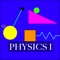# Physics I HD

価格 ｼﾞｬﾝﾙ サイズ このアプリは現在ストアで閲覧することができません。 1800円 ダウンロード教育 38.1MB Andrew Duffy ｼﾞｬﾝﾙ別：---総合：--- 2011-08-02 08:14:05 評価が取得できませんでした。 iOS 8.3以降が必要です。 iPad 対応。 2019-09-18　このアプリは現在ストアから情報が取得できません。削除された可能性があります。
This app covers the Physics that is included in the first semester of a typical introductory college physics course. It is also appropriate for high school physics. The app is essentially an interactive Physics textbook. In addition to standard textbook material, the app also has simulations and animations, as well as links to movies.

The app includes all 15 of the planned 15 chapters. Also included is a bonus chapter from the PhysicsIIHD app, Chapter 21 - Sound and Waves, for anyone who is covering that material in the first semester.

Chapter 1 includes an introduction to basic trigonometry, vectors, and vector addition, all things we will need for the later chapters.

Chapter 2 covers motion in one dimension, going through displacement, velocity, acceleration, and equations we apply in constant-acceleration situations.

Chapter 3 covers forces in one dimension. In this chapter, we discuss what a force is, go over how to draw free-body diagrams, and go through Newton's laws of motion.

Chapter 4 covers two-dimensional motion. Chapter 4 includes sections on relative velocity, the independence of X and Y, and projectile motion, including some example problems.

Chapter 5 covers applications of Newton's laws of motion. The chapter starts with a discussion of friction, both kinetic and static, and it ends by going over uniform circular motion. Also included are sections going over examples of applying Newton's second law, and whole vectors.

Chapters 6 and 7 cover momentum and energy. In chapter 6, we focus on the connections between force and momentum and between force and energy. In chapter 7, we investigate the fundamental ideas of conservation of momentum and conservation of energy.

Chapter 8 covers gravitation in a way that lays the groundwork for the later study of electric charges. Chapter 8 includes Newton's universal law of gravitation, gravitational field, gravitational potential energy, and it concludes by looking at different types of orbits.

Chapter 9, on Fluids, deals with the buoyant force, pressure, and on how to apply energy conservation ideas to flowing fluids. There are also sections on viscosity and surface tension, and drag.

Chapter 10 is the first of two chapters on rotation. The chapter covers rotational kinematics, torque, rotational inertia, and static equilibrium.

In Chapter 11, the emphasis is on rotational dynamics, including rolling without slipping, applying Newton's second law for rotation, angular momentum, and rotational kinetic energy.

Chapter 12, on Simple Harmonic Motion, extends our force ideas and energy ideas to situations involving an object on a spring, and to a pendulum.

Chapter 13 looks at thermal physics from a macroscopic perspective. The chapter covers temperature scales, thermal expansion, specific heat and latent heat, and energy-transfer mechanisms.

Chapter 14 looks at thermal physics from a microscopic perspective. The chapter covers the ideal gas law, kinetic theory (applying basic laws of physics to an ideal gas, leading to a fundamental definition of temperature), the Maxwell-Boltzmann distribution, also introduces the P-V diagram, and discusses diffusion and osmosis.

Chapter 15 covers the laws of thermodynamics. Specifically, the sections included in this update cover the first law, and also go through various thermodynamic processes and a thermodynamic cycle.

In each chapter, the emphasis is on conceptual understanding. Each section of each chapter includes a simulation or animation. Also, in general, each chapter includes over 60 end-of-chapter problems.

Updated to the latest iOS
• 現在ランキング圏外です。ブログパーツ第二弾を公開しました！ホームページでアプリの順位・価格・周辺ランキングをご紹介頂けます。
ブログパーツ第2弾！

アプリの周辺ランキングを表示するブログパーツです。価格・順位共に自動で最新情報に更新されるのでアプリの状態チェックにも最適です。
ランキング圏外の場合でも周辺ランキングの代わりに説明文を表示にするので安心です。

サンプルが気に入りましたら、下に表示されたHTMLタグをそのままページに貼り付けることでご利用頂けます。ただし、一般公開されているページでご使用頂かないと表示されませんのでご注意ください。

オススメ！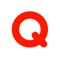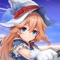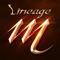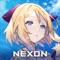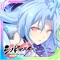お得情報ピックアップ！50%値引中54%値引中54%値引中67%値引中84%値引中「iPhone & iPad アプリランキング」は、最新かつ詳細なアプリ情報をご紹介しているサイトです。 お探しのアプリに出会えるように様々な切り口でページをご用意しております。
メニュー」よりぜひアプリ探しにお役立て下さい。

Presents by \$\$308413110 　　　スマホからのアクセスにはQRコードをご活用ください。 →

Now loading...